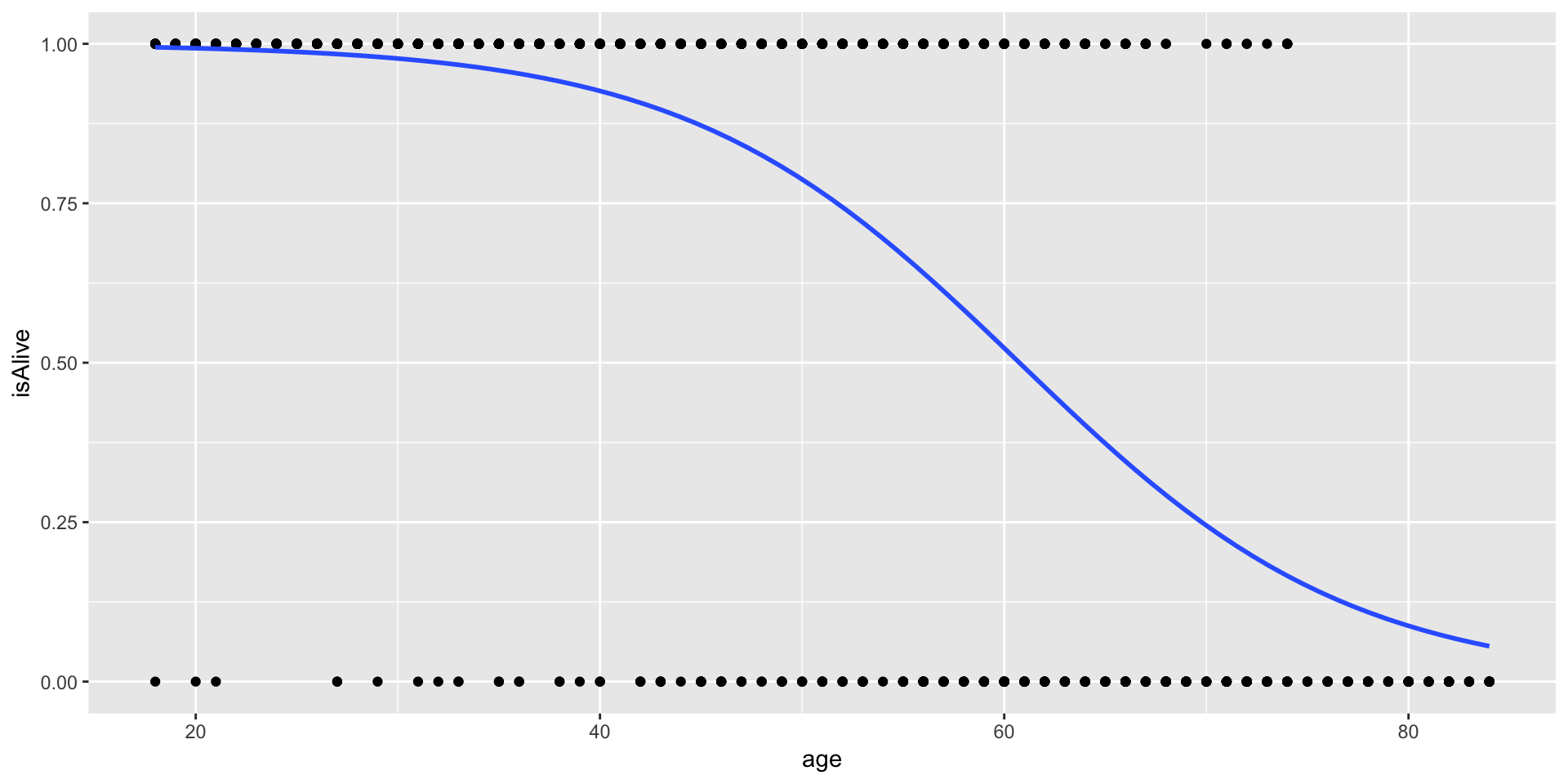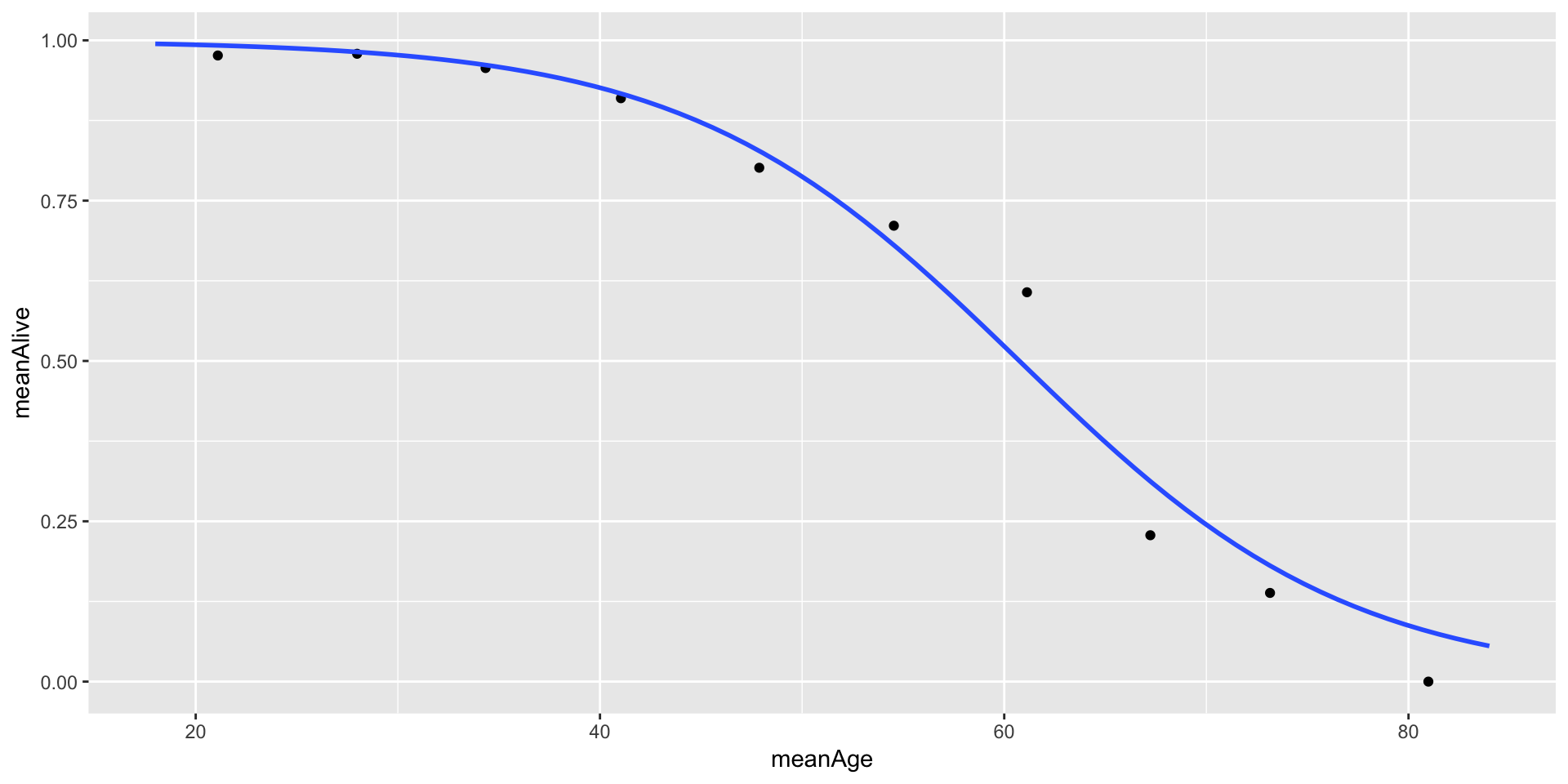library(tidyverse)
library(Stat2Data)
library(skimr)
library(mosaicData)
# install.packages("lmtest")

Often, we want to model a response variable that is binary, meaning that it can take on only two possible outcomes. These outcomes could be labeled “Yes” or “No”, or “True” of “False”, but for all intents and purposes, they can be coded as either 0 or 1. We have seen these types of variables before (as indicator variables), but we always used them as explanatory variables. Creating a model for such a variable as the response requires a more sophisticated technique than ordinary least squares regression. It requires the use of a logistic model.

#### Fitting a Logistic model

For a binary response, instead of modeling $$\pi$$ (the response variable) like this, $\pi = \beta_0 + \beta_1 X$

suppose we modeled it like this, $\log \left( \frac{\pi}{1-\pi} \right) = logit(\pi) = \beta_0 + \beta_1 X$

This transformation is called the logit function. What are the properties of this function? Note that this implies that $\pi = \frac{e^{\beta_0 + \beta_1 X}}{1 + e^{\beta_0 + \beta_1 X}} \in (0,1)$

The logit function constrains the fitted values to line within $$(0,1)$$, which helps to give a natural interpretation as the probability of the response actually being 1.

Fitting a logistic curve is mathematically more complicated than fitting a least squares regression, but the syntax in R is similar, as is the output. The procedure for fitting is called maximum likelihood estimation, and the usual machinery for the sum of squares breaks down. Consequently, there is no notion of $$R^2$$, etc. We use the function glm() (for Generalized Linear Model) to fit a logistic regression.

The data in the Whickham data set (built into mosaicData) contains observations about women, and whether they were alive 20 years after their initial observation. Our goal is to determine how being a smoker affects the probability of being alive, after controlling for age.

data(Whickham)
Whickham <-  Whickham %>%
mutate(isAlive = 2 - as.numeric(outcome))
1. What is this piece of code doing? Why $$2$$?
logm <- glm(isAlive ~ age, data=Whickham, family=binomial)
summary(logm)
##
## Call:
## glm(formula = isAlive ~ age, family = binomial, data = Whickham)
##
## Deviance Residuals:
##     Min       1Q   Median       3Q      Max
## -3.2296  -0.4277   0.2293   0.5538   1.8953
##
## Coefficients:
##              Estimate Std. Error z value Pr(>|z|)
## (Intercept)  7.403126   0.403522   18.35   <2e-16 ***
## age         -0.121861   0.006941  -17.56   <2e-16 ***
## ---
## Signif. codes:  0 '***' 0.001 '**' 0.01 '*' 0.05 '.' 0.1 ' ' 1
##
## (Dispersion parameter for binomial family taken to be 1)
##
##     Null deviance: 1560.32  on 1313  degrees of freedom
## Residual deviance:  946.51  on 1312  degrees of freedom
## AIC: 950.51
##
## Number of Fisher Scoring iterations: 6
ggplot(Whickham, aes(y=isAlive, x=age)) +  geom_point() +
geom_smooth(method = "glm",  method.args = list(family = "binomial"), se = FALSE)1. How can we interpret the coefficients of this model in the context of the problem?
logm2 <- glm(isAlive ~ age + smoker, data=Whickham, family=binomial)
summary(logm)
##
## Call:
## glm(formula = isAlive ~ age, family = binomial, data = Whickham)
##
## Deviance Residuals:
##     Min       1Q   Median       3Q      Max
## -3.2296  -0.4277   0.2293   0.5538   1.8953
##
## Coefficients:
##              Estimate Std. Error z value Pr(>|z|)
## (Intercept)  7.403126   0.403522   18.35   <2e-16 ***
## age         -0.121861   0.006941  -17.56   <2e-16 ***
## ---
## Signif. codes:  0 '***' 0.001 '**' 0.01 '*' 0.05 '.' 0.1 ' ' 1
##
## (Dispersion parameter for binomial family taken to be 1)
##
##     Null deviance: 1560.32  on 1313  degrees of freedom
## Residual deviance:  946.51  on 1312  degrees of freedom
## AIC: 950.51
##
## Number of Fisher Scoring iterations: 6
1. How does the smoker term affect the model?
##### Binning

Another way to make sense of a binary response variable is to bin the explanatory variable and then compute the average proportion of the response within each bin.

Whickham <- Whickham %>%
mutate(ageGroup = cut(age, breaks=10))
Whickham %>%
group_by(ageGroup) %>%
skim(isAlive)
## Skim summary statistics
##  n obs: 1314
##  n variables: 5
##  group variables: ageGroup
##
## ── Variable type:numeric ───────────────────────────────────────────────────────────────────────────────────────────────────
##     ageGroup variable missing complete   n mean   sd p0 p25 p50 p75 p100
##  (17.9,24.6]  isAlive       0      127 127 0.98 0.15  0   1   1   1    1
##  (24.6,31.2]  isAlive       0      191 191 0.98 0.14  0   1   1   1    1
##  (31.2,37.8]  isAlive       0      162 162 0.96 0.2   0   1   1   1    1
##  (37.8,44.4]  isAlive       0      144 144 0.91 0.29  0   1   1   1    1
##    (44.4,51]  isAlive       0      151 151 0.8  0.4   0   1   1   1    1
##    (51,57.6]  isAlive       0      128 128 0.71 0.46  0   0   1   1    1
##  (57.6,64.2]  isAlive       0      168 168 0.61 0.49  0   0   1   1    1
##  (64.2,70.8]  isAlive       0       92  92 0.23 0.42  0   0   0   0    1
##  (70.8,77.4]  isAlive       0       94  94 0.14 0.35  0   0   0   0    1
##  (77.4,84.1]  isAlive       0       57  57 0    0     0   0   0   0    0
##      hist
##  ▁▁▁▁▁▁▁▇
##  ▁▁▁▁▁▁▁▇
##  ▁▁▁▁▁▁▁▇
##  ▁▁▁▁▁▁▁▇
##  ▂▁▁▁▁▁▁▇
##  ▃▁▁▁▁▁▁▇
##  ▅▁▁▁▁▁▁▇
##  ▇▁▁▁▁▁▁▂
##  ▇▁▁▁▁▁▁▁
##  ▁▁▁▇▁▁▁▁

Although this is not the preferred method for performing logistic regression, it can be illustrative to see how the logistic curve fits through this series of points.

binned <- Whickham %>%
group_by(ageGroup) %>%
dplyr::summarize(meanAlive = mean(isAlive), meanAge = mean(age))

ggplot() + geom_point(data=binned, aes(x=meanAge, y=meanAlive)) + geom_smooth(data=Whickham, aes(x=age, y=isAlive), method='glm', method.args = list(family = "binomial"), se = FALSE)